11/05/2020

## Seed used in these slides

set.seed(1024)

## Libraries used in these slides

library(rpart)
library(rpart.plot)
library(mlbench)
library(DMwR2)
library(e1071)

## Properties

• Interpretable results
• Reasonable accuracy
• Applicable for both classification and regression tasks
• Works with both numeric and categorical variables
• Can handle NAs
• No assumption of the shape of the function
• Not top prediction performance
• Ensembles of trees have much better performance

## Shape

• A hierarchy of logical tests on variables
• Is X > 5?
• Is color = green?
• Is birthplace in {Ankara, Istanbul, İzmir}?
• Each branch, including the root splits the data at hand into two
• Decreasing the total error rate
• The leaves contain results / predictions
• The path to a leaf is a conjunction of logical tests

## Example

##   "Id"              "Cl.thickness"    "Cell.size"       "Cell.shape"
##   "Marg.adhesion"   "Epith.c.size"    "Bare.nuclei"     "Bl.cromatin"
##   "Normal.nucleoli" "Mitoses"         "Class"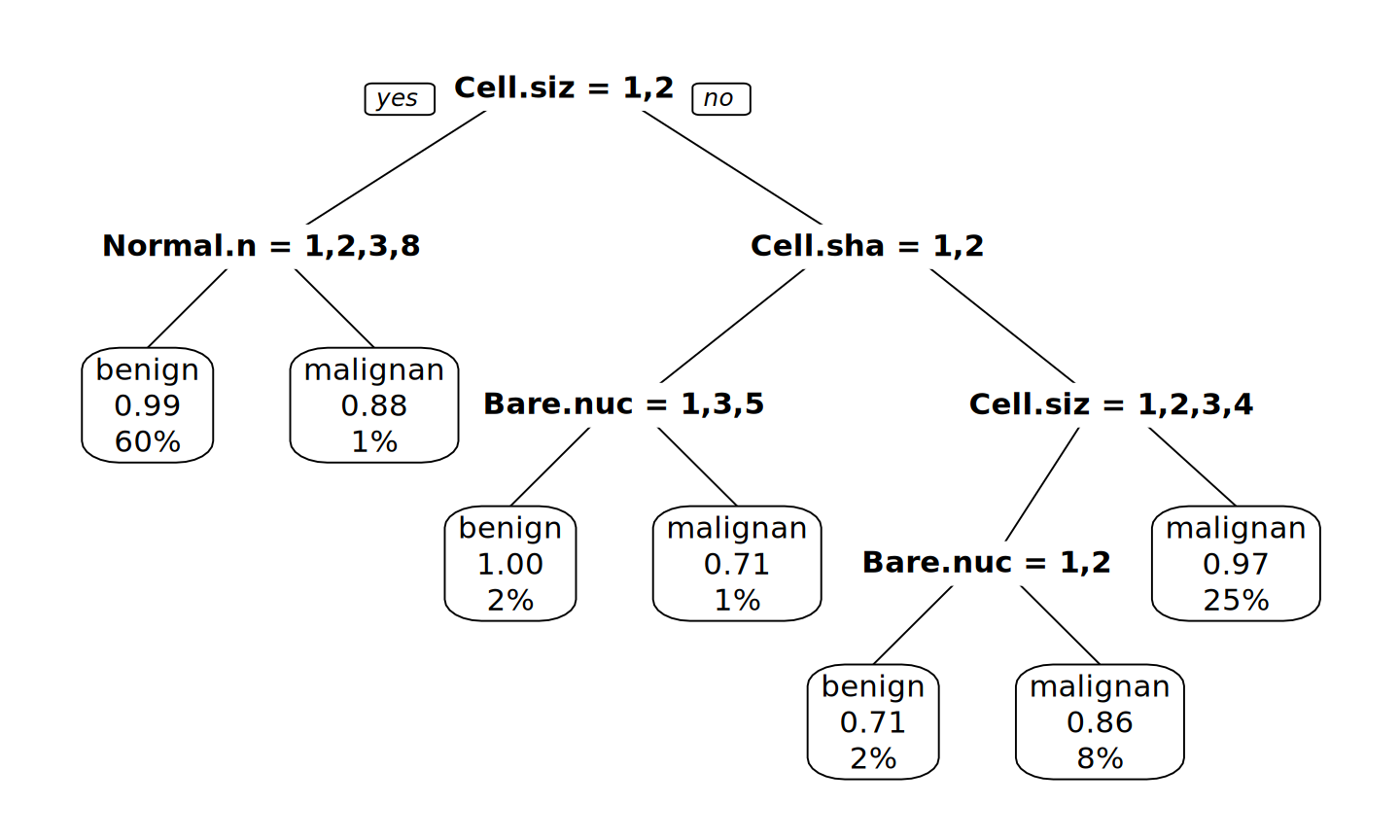## Algorithm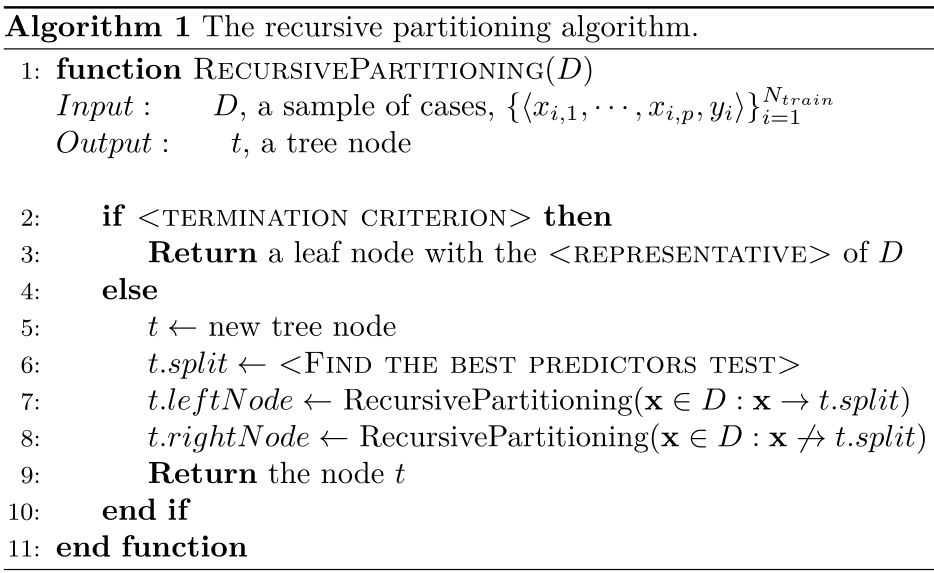The recursive partitioning algorithm

## Find Best Split: GINI index

The Gini index of a dataset D, where each example belongs to one of C classes:

$$\displaystyle Gini(D) = 1 - \sum_{i=1}^{C}{p_i^2}$$

• $$p_i$$ is the observed frequency of class i.
• Consider a binary case where two classes are A and B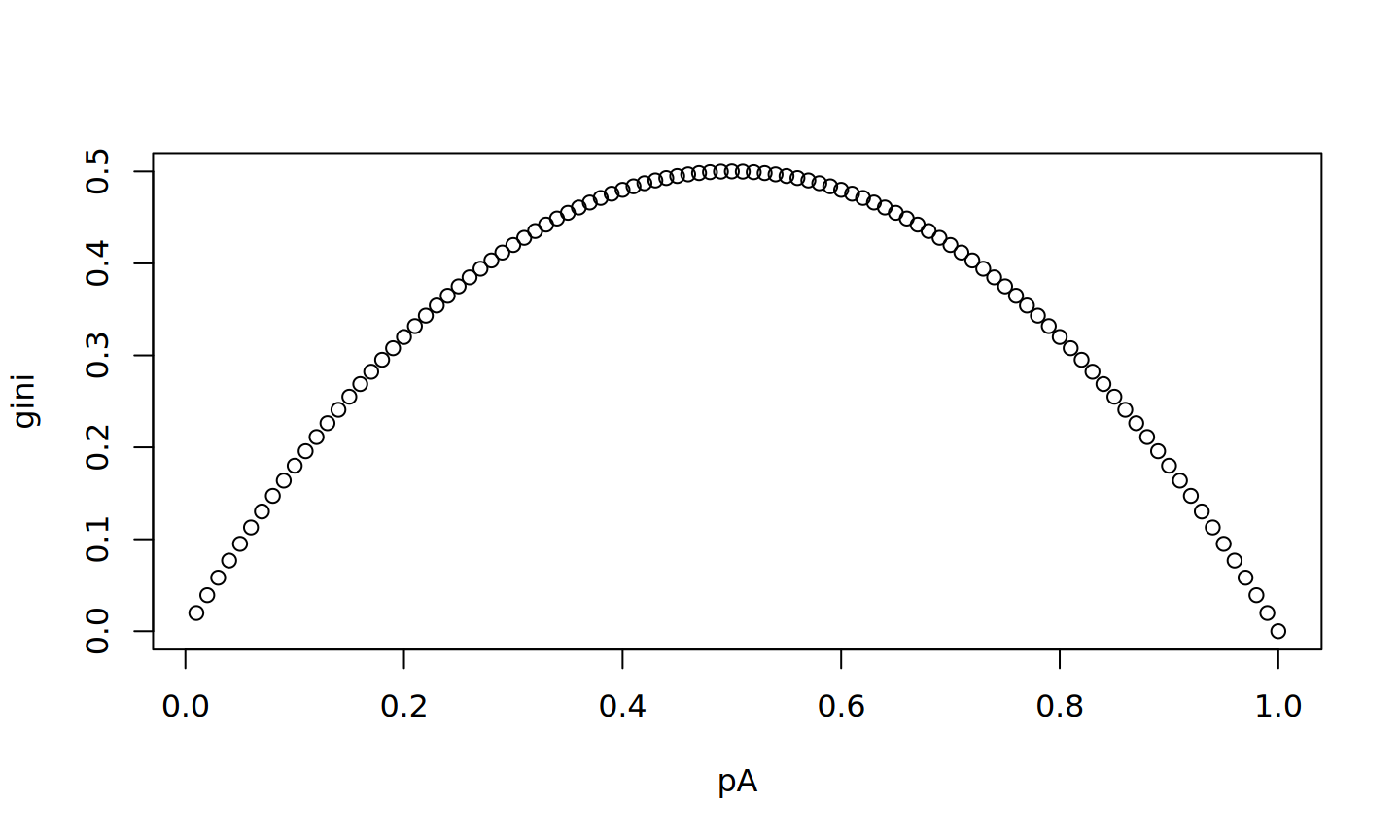## GINI index

If D is split by a logical test s, then

$$\displaystyle Gini_s(D) = \frac{|D_s|}{|D|}Gini(D_s) + \frac{|D_{\neg s}|}{|D|}Gini(D_{\neg s})$$

Then, the reduction in impurity is given by

$$\Delta Gini_s(D) = Gini(D) - Gini_s(D)$$

• Information gain based on entropy is also frequently used

## Least Squares

• For regression, LS is frequently used to measure error

$$\displaystyle Err(D) = \frac{1}{|D|} \sum_{ \langle x_i,y_i \rangle \in D}{(y_i - k_D)^2}$$

where $$k_D$$ is the constant representing value of D.

• It is shown that $$mean(y_i)$$ actually minimizes LS.

• If D is split by a logical test s, then

$$\displaystyle Err_s(D) = \frac{|D_s|}{|D|}Err(D_s) + \frac{|D_{\neg s}|}{|D|}Err(D_{\neg s})$$

Then, the reduction in impurity is given by

$$\Delta Err_s(D) = Err(D) - Err_s(D)$$

## Termination

• When to stop?
• Too deep -> over-fitting, variance error
• Too shallow -> over-simplified, bias error
• Control with parameters
• leaf size
• split size
• depth
• complexity
• Grow a very large tree, then prune
• According to some statistical information

## Implementation

• Implemented in rpart and party
• We will use rpart
• Functions
• rpart() and prune.rpart()
• Book package contains
• rpartXse() which combines rpart() and prune.rpart()
• applies post-prunning with X-SE rule

## Formula

• A formula in R is provided in the following form

$$Y \sim X_1 + X_2 + X_3 + X_4...$$

• This means the value of Y depends on the values of Xs

$$Y \sim .$$

• means Y vs. everything else

## Randomicity

• Due to the certain randomized parts of the algorithm, it is possible to obtain slightly different trees between different runs.

• Hence, always use a seed

• rpart.plot package allows nice drawings of DTs using prp

## Example

data(iris)
ct1 <- rpartXse(Species ~ ., iris, model = TRUE)
ct2 <- rpartXse(Species ~ ., iris, se = 0, model = TRUE)
• se=0 is a less agressive prunning

## Example

par(mfrow=c(1,2))
prp(ct1, type = 0, extra = 101)
prp(ct2, type = 0, extra = 101)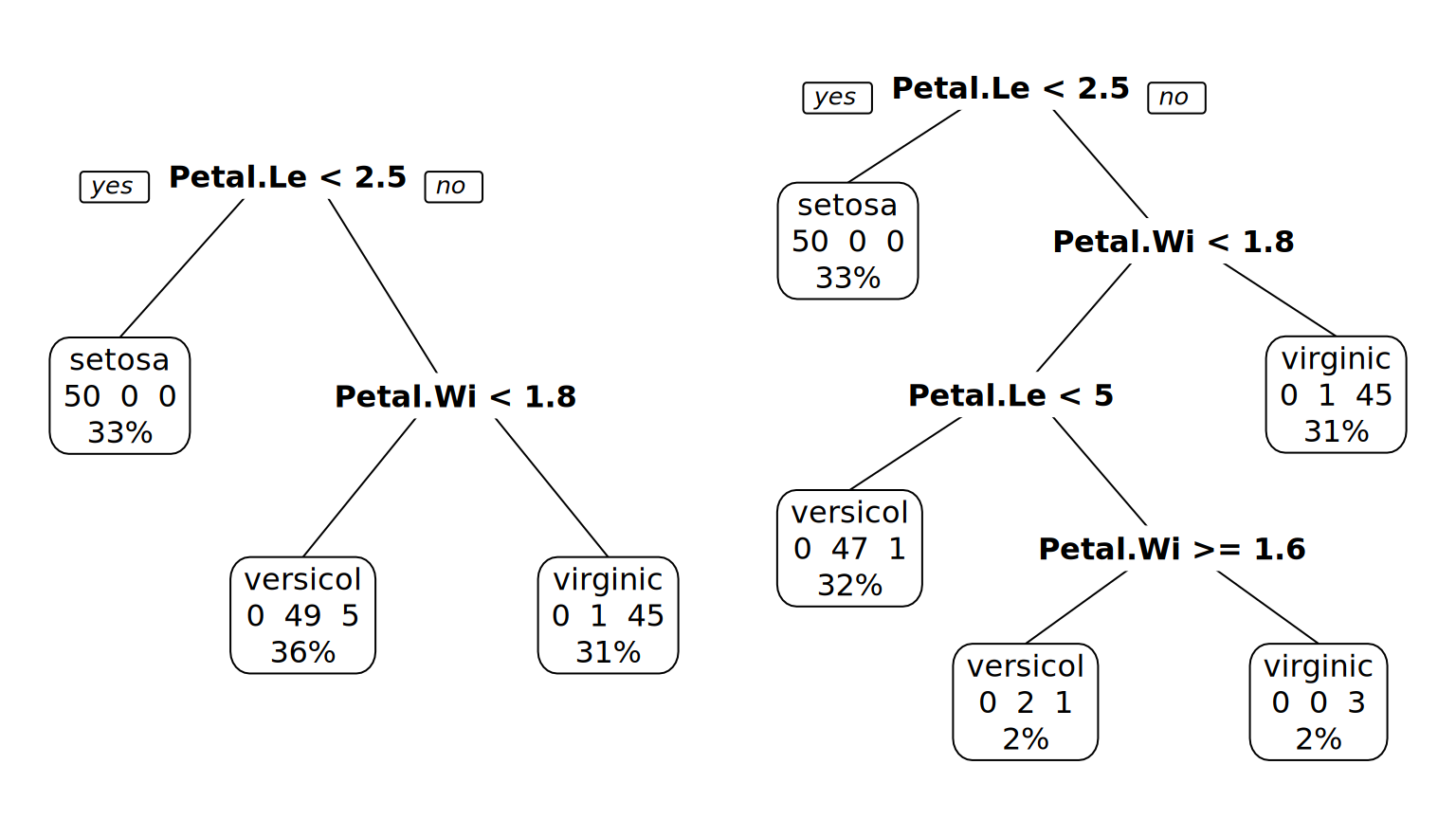## Example

samp <- sample(1:nrow(iris), 120)
tr_set <- iris[samp, ]
tst_set <- iris[-samp, ]
model <- rpartXse(Species ~ ., tr_set, se = 0.5)
predicted <- predict(model, tst_set, type = "class")
head(predicted)
##     12     15     35     37     40     43
## setosa setosa setosa setosa setosa setosa
## Levels: setosa versicolor virginica
table(tst_set$Species, predicted) ## predicted ## setosa versicolor virginica ## setosa 8 0 0 ## versicolor 0 10 1 ## virginica 0 0 11 errorRate <- sum(predicted != tst_set$Species) / nrow(tst_set)
errorRate
##  0.03333333

## Support Vector Machines

• Linearly separable sets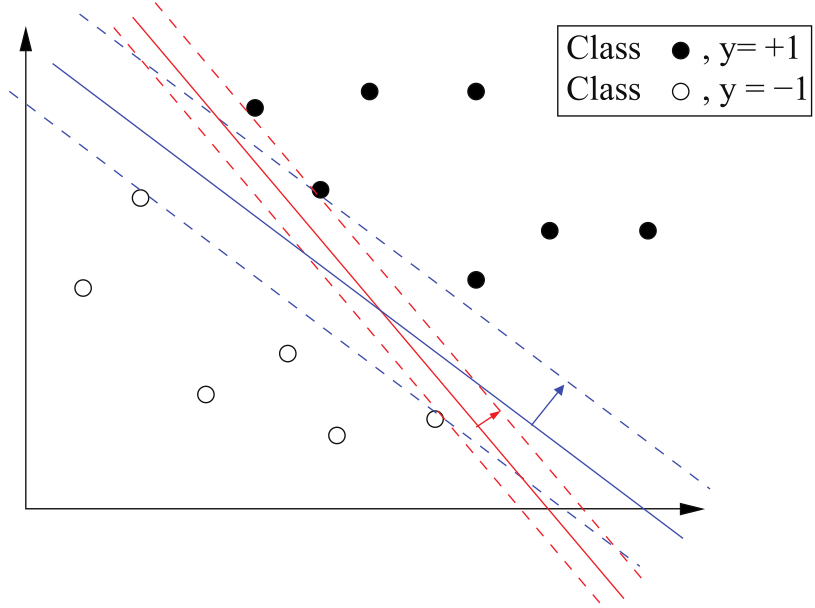## Support Vector Machines

• Linearly non-separable sets
• Lift to a higher dimension using a non-linear function